# Equilibrium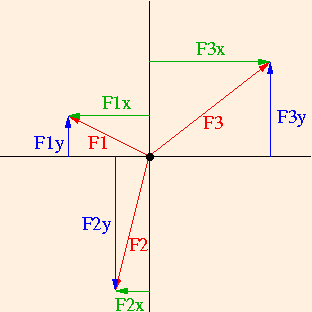F1x + F2x = F3x.
Similarly, the sum of the forces in the y direction will be zero if the sum of the magnitudes of the forces pulling up are equal to the sum of the magnitudes of the forces pulling down:
F1y + F3y = F2y.
Using the capital Greek letter sigma to denote summation, we have
ΣFleft = ΣFright and

ΣFup = ΣFdown.

If these conditions are met, the center of mass of this system will not accelerate in either the x or y directions. But what about rotation?

Consider a one-dimensional object lying in a plane with forces acting on it has shown: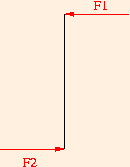Since there are no forces acting in the y direction, and the sum of the forces acting in the x direction are zero, the center of mass of the object will not move. It is clear however, that the object will rotate about its own center: the torques due to both forces result in rotation in the counterclockwise direction. So we see that in two dimensions, there are actually three conditions for equilibrium:

ΣFleft = ΣFright,

ΣFup = ΣFdown and

Σ τcounterclockwise = Σ τclockwise.

The first two conditions provide for translational equilibrium: the center of mass of the system will not move either left or right or up or down, and the final condition provides for rotational equilibrium: the system will not rotate about its own center of mass.

### Skeletomuscular Equilibrium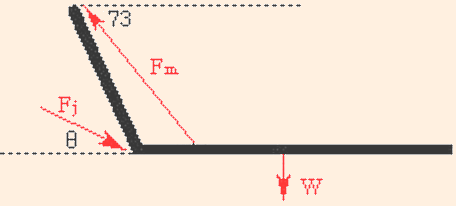We wish to find the forces required to hold the arm in place. The biceps muscle pulls upward with a tension (force) Fm, and the upper arm exerts a force Fj at the elbow joint, on the lower arm to keep it in place. For the purposes of this example, we will assume that the biceps muscle is attached to the arm 4 cm from the elbow, and that the lower arm is 40 cm long. The mass of the lower arm is assumed to be 14 kg; this causes a force W which is exerted downward by gravity at its center of mass. This is at its midpoint, since it is assumed to be a uniform rod.

Our first step is to identify the x and y components of each force: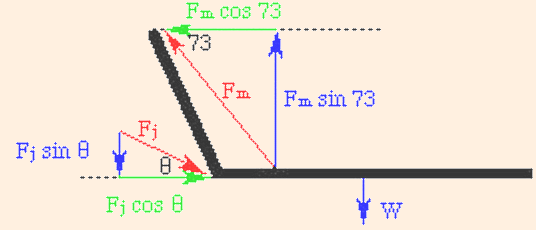Note that we do not need to do this for W; it is already vertical, and has no horizontal component. Using standard right triangle trigonometry, the x and y equations are:

Fm cos 73 = Fj cos θ

and

Fm sin 73 = W + Fj sin θ,

where W = m g.

The next step is to choose a pivot for the torque equations, and identify the force components which are perpendicular to the lever arm. For this system the lower arm is the lever arm, and we choose the elbow for the pivot. Since Fj acts at the pivot, its lever arm (distance from the pivot) is zero, and hence so is its torque. The components of the forces which are perpendicular to the lever arm are Fmy and W, since in this example the lever arm is horizontal. Since the lever arm for the bicep is 4 cm and the lever arm for the weight W is halfway up the 40 cm arm, the torque equation is:

4 Fm sin 73 = (40 / 2) W.

From it we obtain Fm = 717 N. Note that in the torque equation, we don't have to convert the lever arm lengths to meters; it is only necessary that the units match on both sides. Subtracting W from both sides of the y equation and dividing it by the equation for x, we obtain

tan θ = (Fm sin 73 - W) / Fm cos 73.

This gives us a value of 69 degrees for θ, and 587 N for Fj.

Now consider the compression of the vertabrae in the spine which occurs when a person leans over: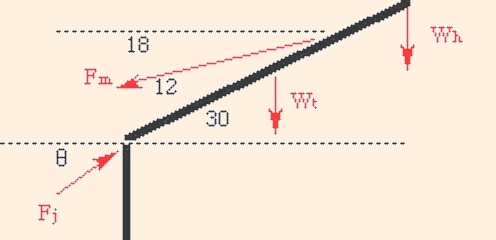For this example, we will assume that the muscle is attached two thirds of the way up from the bottom of the spine; the mass of the head and arms is 16 kg, and the mass of the torso is 32 kg. Note that in this case, the weight of the torso is applied at the center of mass, and the head is treated as a point mass at the end of the torso. Our first step is to identify the x and y components of the forces: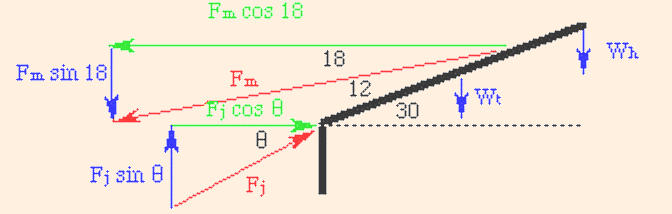Here we have exaggerated the forces in order to clarify the drawing. The x and y equations for this problem are:

Fm cos 18 = Fj cos θ

and

Fj sin θ = Wh + Wt + Fm sin 18.

The next step is to find the components of the forces which are perpendicular to the lever arm. The lever arm is the spine, and the pivot is chosen at the base of the spine to eliminate Fj from the torque equation. We now draw triangles (again exaggerating the forces) whose hypotenuse are the forces and whose sides are perpendicular and parallel to the lever arm: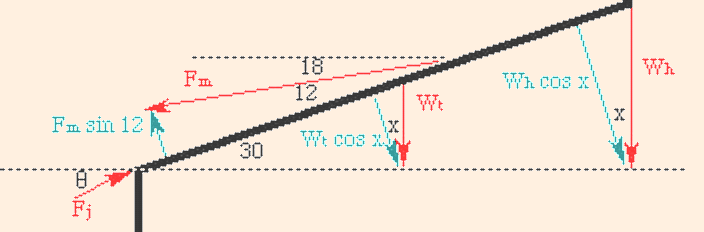At this point we don't know the angle x, but we do know that the triangles with the vertical weights are similar. Consider the following triangles: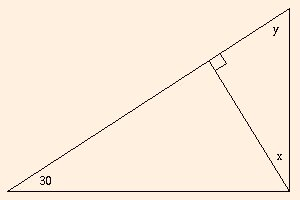Since the sum of the angles in a triangle is 180 degrees, and these are right triangles, we know that

y + 30 = 90

and

x + y = 90.

This implies that x = 30 degrees. Since the muscle acts two thirds of the way up the spine, that is its lever arm; the lever arm for the weight of the torso is at its center of mass in the middle of the spine, and that for the head is at the neck end of the spine. The torque equation is then

(2 L / 3) Fm sin 12 = L Wh cos 30 + (L / 2) Wt cos 30,

where L is the length of the spine. Note that since it appears as a factor in every term in the equation for torque, the length of the spine is not important in this problem. Note also that the angle in the torque equation is different from that in the x and y equations. This is because the torque is computed from the component of the force which is perpendicular to the lever arm, which is this example is not the y component! The solution for this problem is

Fm = 1959 N,

Fj = 2151 N and

θ = 30 degrees.

If the person is picking up a 10 kg mass, Wh is increased by 980 N and the new results are
Fm = 2572 N,

Fj = 2800 N and

θ = 29.1 degrees.

Compare this result with that from the previous example (particularly the values of Fj), and you will understand why lower back pain is such a common malady!

You need a Java-capable browser to be able to use the applets. If they do not work with your Windows system, download the Java VM (Virtual Machine) for your version of Windows at the download section at java.sun.com.

The kind of equilibrium described in this section is often called "statics", because all forces cancel and nothing can undergo a change in velocity; so if all velocities start out at zero, they remain there. The skeletomuscular problems we examine are statics problems from a physical point of view, even though the muscles are continually burning calories to maintain the static configuration. So from a biochemical point of view, the system is not in equilibrium, while from a physical point of view it is.

The next section begins our work with thermal physics.